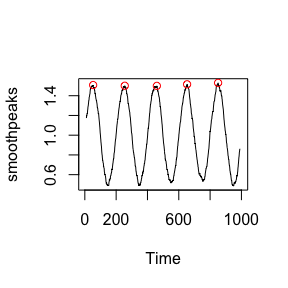Introduction

There is no panacea for detecting peaks in one-dimensional series of data, but there are algorithms that have been proven to work well in practice in certain settings. This package implements 2 heuristics motivated by genomics data analysis.

The first algorithm (detect.spikes) detects brief spikes rising above a user-defined number of standard deviations computed over widows, whose length the user also defines. The algorithm is iterative: Detected spikes are excluded from standard deviation estimations, and another detection round begins. The algorithm stops when the number of detected peaks does not change significantly.

The second algorithm (peakpick) detects smooth peaks in data that should probably be smoothed or filtered before. This algorithm tries to estimate peaks via derivatives, and additionally requires the peaks to rise a user-defined number of standard deviations above their vicinity. The user can also specify that the peaks must lie a certain number of data points apart.

The algorithms were designed by Weber et al., the full reference is given below.

Examples

The library is loaded like so:

require(peakPick)

To demonstrate spike detection, we generate two sets of uniformly distributed data, each with 100 points. The sets must be ordered columnwise. Spikes are then introduced at positions 40 and 50, respectively.

set.seed(123)
spikes <- matrix(runif(200), ncol=2)
spikes[40, 1] <- 300
spikes[50, 2] <- 40

We use a window of +/- 10 positions to detect spikes in these data; we set a limit of 12 standard deviations to avoid detecting spurious peaks.

spikehits <- detect.spikes(spikes, c(11, 90), 10, spike.min.sd=12)

## Spikes are correctly recovered
which(spikehits[, 1])
##  40
which(spikehits[, 2])
##  50

To demonstrate smooth peak detection, we generate a series of noisy oscillations, which we filter by means of running averages (this will generate a time series object):

set.seed(123)
peaks <- 1 + 0.5*sin((1:1000)*pi/100) + 0.1*rnorm(1000)
smoothpeaks <- filter(peaks, rep(1/20, 20), sides=2)

Peaks are now readily detected; data must be supplied as a matrix with the series organized columnwise. We set peak.npos to reflect our expectation of peak broadness. This parameter defines the window length used for mean and standard deviation estimations; peaks must rise a certain multiple of standard deviation above mean. Note that results are given as boolean vectors.

peakhits <- peakpick(matrix(smoothpeaks, ncol=1), 100, peak.npos=50)

## Plot results
plot(smoothpeaks)
points((1:1000)[peakhits], smoothpeaks[peakhits], col="red")